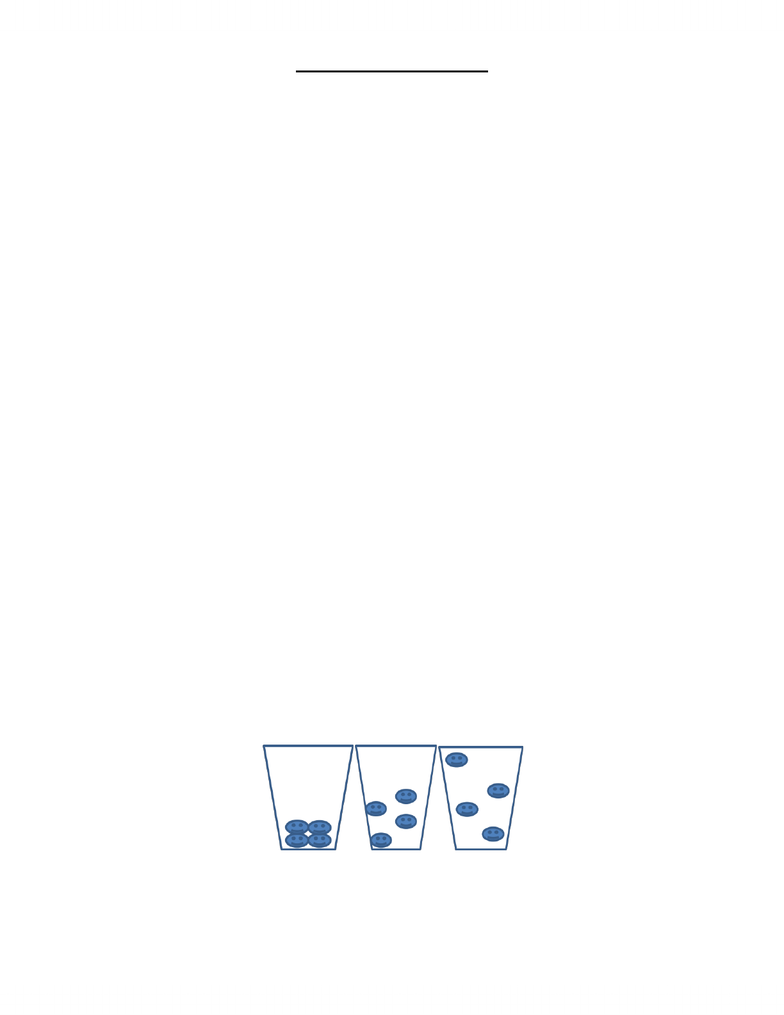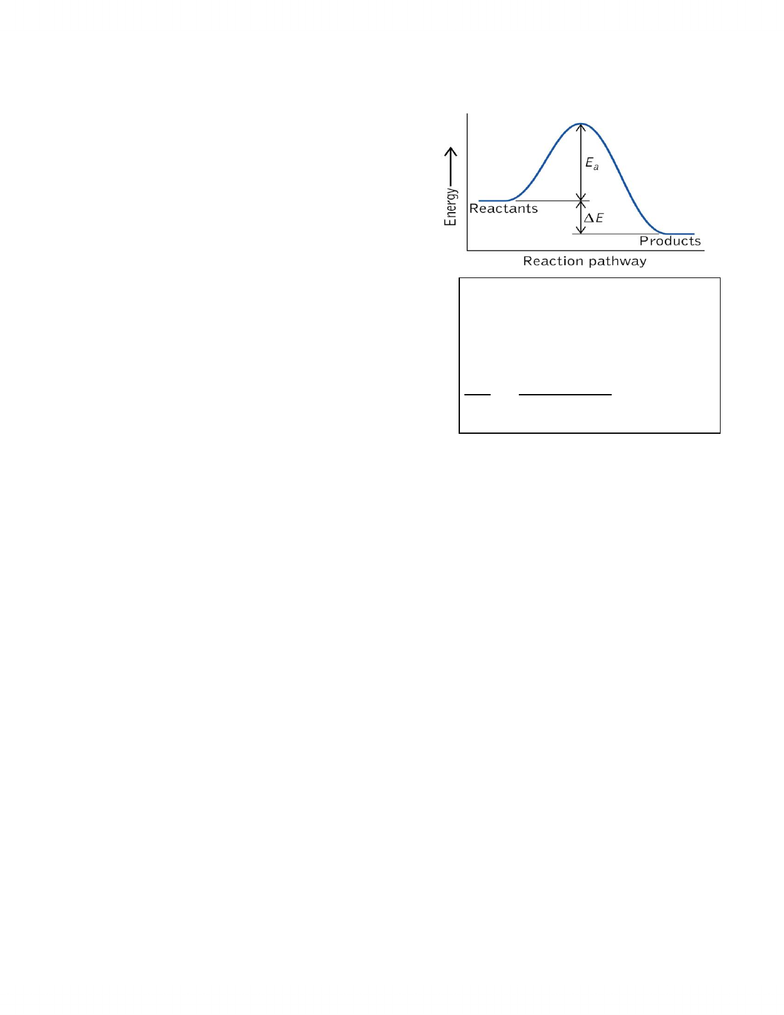Study Guides (390,000)
CA (150,000)
UOIT (400)
CHEM (10)
Final

CHEM 1020U Study Guide - Final Guide: Kelvin, Trigonal Planar Molecular Geometry, Thermodynamics

School
UOIT
Department
Chemistry
Course Code
CHEM 1020U
Professor
Omer Yukseker
Study Guide
Final

This preview shows pages 1-3. to view the full 11 pages of the document.Chemistry II Exam Review
Part One: Thermodynamics
1. Thermodynamics so far
- Conservation of Energy: Energy cannot be created or destroyed. It can only be converted from one
form to another.
- State Function: function or property whose value depends on the present state or condition of the
system, not the pat used to arrive at that state.
2. First Law of Thermodynamics
- Energy is conserved, not created
3. Enthalpy (H)
- H = E + PV
- At constant pressure, the enthalpy change is equal to the heat, ΔH = qp
4. Second Law of Thermodynamics
- The entropy of the universe always increases
5. Entropy (s)
- The measure of the amount of disorder in a system
- ΔSuniverse = ΔSsystem + ΔSsurroundings > 0
- This determines which reactions will occur
- If ΔSsystem < 0 for a reaction, the value of ΔSsurroundings must be positive, and be larger than ΔSsystem
- The reaction will NOT occur if this is the case
- ΔS = ΣSproducts ΣSreactants

Only pages 1-3 are available for preview. Some parts have been intentionally blurred.6. Third Law of Thermodynamics
- A perfect crystal at zero kelvin is assigned an entropy of zero.
- Suggests that there is two sources of disorder in a system:
- i. A perfect crystal
- ii. At zero Kelvin
- Perfect Crystal: there is disorder due to the positions of atoms, molecules, etc.
- Ssolid < Sliquid <<<< Sgas
- At zero kelvin: there is disorder due to the movement of atoms, molecules etc.
- In a given phase, the entropy will go up with T.
- Kelvin scale: it starts at absolute zero below which there is no colder temperature than 0K
7. Gibb’s Free Energy
- G ≡ H – TS
- ΔG = ΔH Δ(TS)
- For Isothermal reactions we use: ΔG = ΔH TΔS (at constant temperature)
- At constant T, P, the sign of ΔG tells us whether a reaction is spontaneous or not.
- Until now, the only way to determine spontaneity was to calculate Δsuniverse.
- Calculating ΔG is much easier, since its value depends only on the system.
- As the temperature increases, the reaction will go from spontaneous to not so spontaneous
- ΔG°rxn = ΣΔG°(products) - ΣΔG°(reactants)
ΔH
ΔS
ΔG
Spontaneity (Constant T and P)
<0
>0
Always <0
Always spontaneous
<0
<0
<0 at low temperature
Spontaneous at low
temperatures
>0
>0
<0 at high temperature
Spontaneous at high
temperatures
>0
<0
Always >0
Never spontaneous

Only pages 1-3 are available for preview. Some parts have been intentionally blurred.Part Three: Equilibrium
1. Chemical Equilibrium
- We saw in kinetics that the rate of a reaction
depended on the height of the energy barrier (Ea)
- A larger Ea means a slower reaction, since a lower
percentage of all molecules will have enough
energy to overcome the barrier
- What about the reverse reaction?
- products reactants
- The activation energy for the reverse reaction (Ea,r) is
larger than the activation energy for the forward
reaction
- the reverse reaction should occur more slowly
- Until now we have only considered reactions where
Ea,r is so large that the effective rate of the reverse
reaction is zero
- rateforward = kforward[A]1
- ratereverse = kreverse[B]1
- When the forward and reverse reactions happen at the same rate, we have reached CHEMICAL
EQUILIBRIUM
- The concentrations of chemicals do not change
- their rates of production and loss are equal
- since the chemistry (the reactions) are ongoing (they don’t stop), this is called DYNAMIC
EQUILIBRIUM
- aA + bB <- - > cC + dD
- the forward rate = kf[A]a[B]b
- the reverse rate = kr[C]c[D]d
2. Aspects of Equilibrium States
- They display no macroscopic evidence of change
- They are reached through spontaneous processes
- They show a dynamic of forward and reverse processes
- They are the same regardless of direction of approach
K
k
k
kk
ba
dc
r
f
dc
r
ba
f
reverseforward
B][A][
D][C][
D][C][B][A][
raterate
You're Reading a Preview

Unlock to view full version